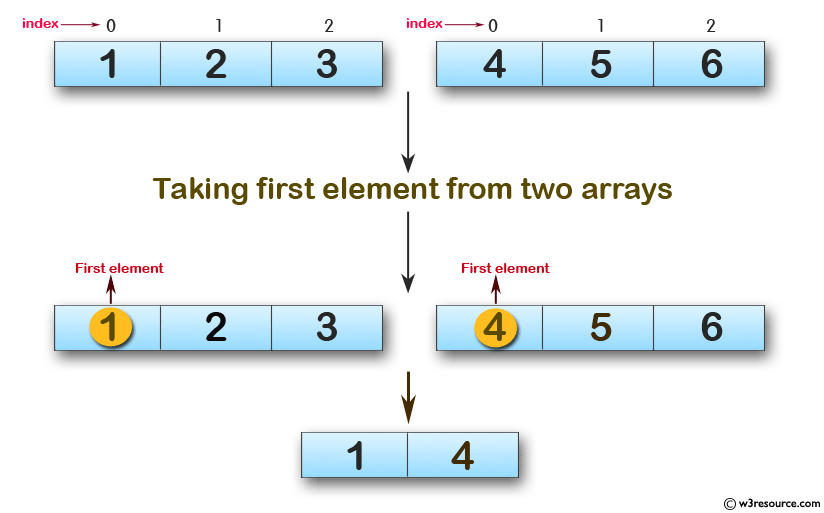﻿ Swift Array Programming Exercise: Create a new array taking the first element from two given arrays of integers - w3resource# Swift Array Programming Exercises: Create a new array taking the first element from two given arrays of integers

## Swift Array Programming: Exercise-23 with Solution

Write a Swift program to create a new array taking the first element from two given arrays of integers. If either array is length 0, ignore that array.

Pictorial Presentation:Sample Solution:

Swift Code:

``````func new_array(_ array1: [Int], _ array2: [Int]) -> [Int] {

if array1.count == 0 && array2.count == 0
{
return []
}
else if array1.count == 0 && array2.count != 0
{
return [array2.first!]
}
else if array1.count != 0 && array2.count == 0
{
return [array1.first!]
}
else
{
return [array1.first!, array2.first!]
}
}

print(new_array([1, 2, 3], [4, 5, 6]))
print(new_array([], []))
print(new_array(, ))
print(new_array([1, 5], []))
```
```

Sample Output:

```[1, 4]
[]
[1, 2]

```

Swift Programming Code Editor:

Improve this sample solution and post your code through Disqus

What is the difficulty level of this exercise?

﻿